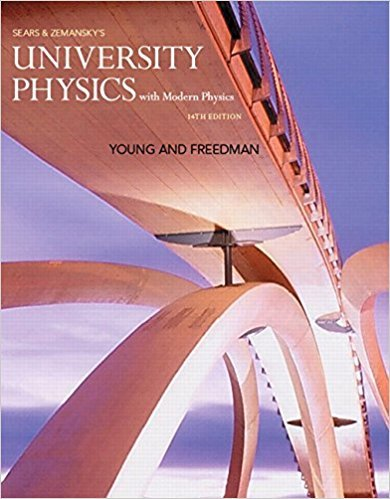×
Get Full Access to University Physics With Modern Physics (1) - 14 Edition - Chapter 17 - Problem 17.118
Get Full Access to University Physics With Modern Physics (1) - 14 Edition - Chapter 17 - Problem 17.118

×

# In another experiment, you place a layer of thisISBN: 9780321973610 228

## Solution for problem 17.118 Chapter 17

University Physics with Modern Physics (1) | 14th Edition

• Textbook Solutions
• 2901 Step-by-step solutions solved by professors and subject experts
• Get 24/7 help from StudySoup virtual teaching assistantsUniversity Physics with Modern Physics (1) | 14th Edition

4 5 1 408 Reviews
13
0
Problem 17.118

In another experiment, you place a layer of this cryoprotectant between one 10 cm * 10 cm cold plate maintained at -40C and a second cold plate of the same size maintained at liquid nitrogens boiling temperature (77 K). Then you measure the rate of heat transfer. Another lab wants to repeat the experiment but uses cold plates that are 20 cm * 20 cm, with one at -40C and the other at 77 K. How thick does the layer of cryoprotectant have to be so that the rate of heat transfer by conduction is the same as that when you use the smaller plates? (a) One-quarter the thickness; (b) half the thickness; (c) twice the thickness; (d) four times the thickness.

Step-by-Step Solution:
Step 1 of 3

Chapter 2: Simple Harmonic Motion 2.1 The Simplest Vibration 1. What is the simplest possible system that demonstrates vibration a. A mass suspended on a spring demonstrates our system at rest: the mass is at the equilibrium position, and the spring is (somehow) stretched, also in it equilibrium position. i. The force of gravity balances the elastic force from a spring. ii. This elastic force depends on the elongation of our spring: 1. F = ­kx a. x is elongation and k is stiffness b. k depends on thickness of the wire as well as on the material our spring is made of. c. The minus sign demonstrates that elastic force is always directed against elongation (squeezing), trying to bring the suspended mass back to the equilibrium point. i. This kind of force is called restoring force, and its presences is necessary

Step 2 of 3

Step 3 of 3

##### ISBN: 9780321973610

Since the solution to 17.118 from 17 chapter was answered, more than 232 students have viewed the full step-by-step answer. The answer to “In another experiment, you place a layer of this cryoprotectant between one 10 cm * 10 cm cold plate maintained at -40C and a second cold plate of the same size maintained at liquid nitrogens boiling temperature (77 K). Then you measure the rate of heat transfer. Another lab wants to repeat the experiment but uses cold plates that are 20 cm * 20 cm, with one at -40C and the other at 77 K. How thick does the layer of cryoprotectant have to be so that the rate of heat transfer by conduction is the same as that when you use the smaller plates? (a) One-quarter the thickness; (b) half the thickness; (c) twice the thickness; (d) four times the thickness.” is broken down into a number of easy to follow steps, and 122 words. This full solution covers the following key subjects: . This expansive textbook survival guide covers 44 chapters, and 4574 solutions. This textbook survival guide was created for the textbook: University Physics with Modern Physics (1), edition: 14. University Physics with Modern Physics (1) was written by and is associated to the ISBN: 9780321973610. The full step-by-step solution to problem: 17.118 from chapter: 17 was answered by , our top Physics solution expert on 01/09/18, 07:46PM.

## Discover and learn what students are asking

Statistics: Informed Decisions Using Data : The Normal Approximation to the Binomial Probability Distribution
?In a binomial experiment with n trials and probability of success p, if ________, the binomial random variable X is approximately normal with ?x= and

Unlock Textbook Solution